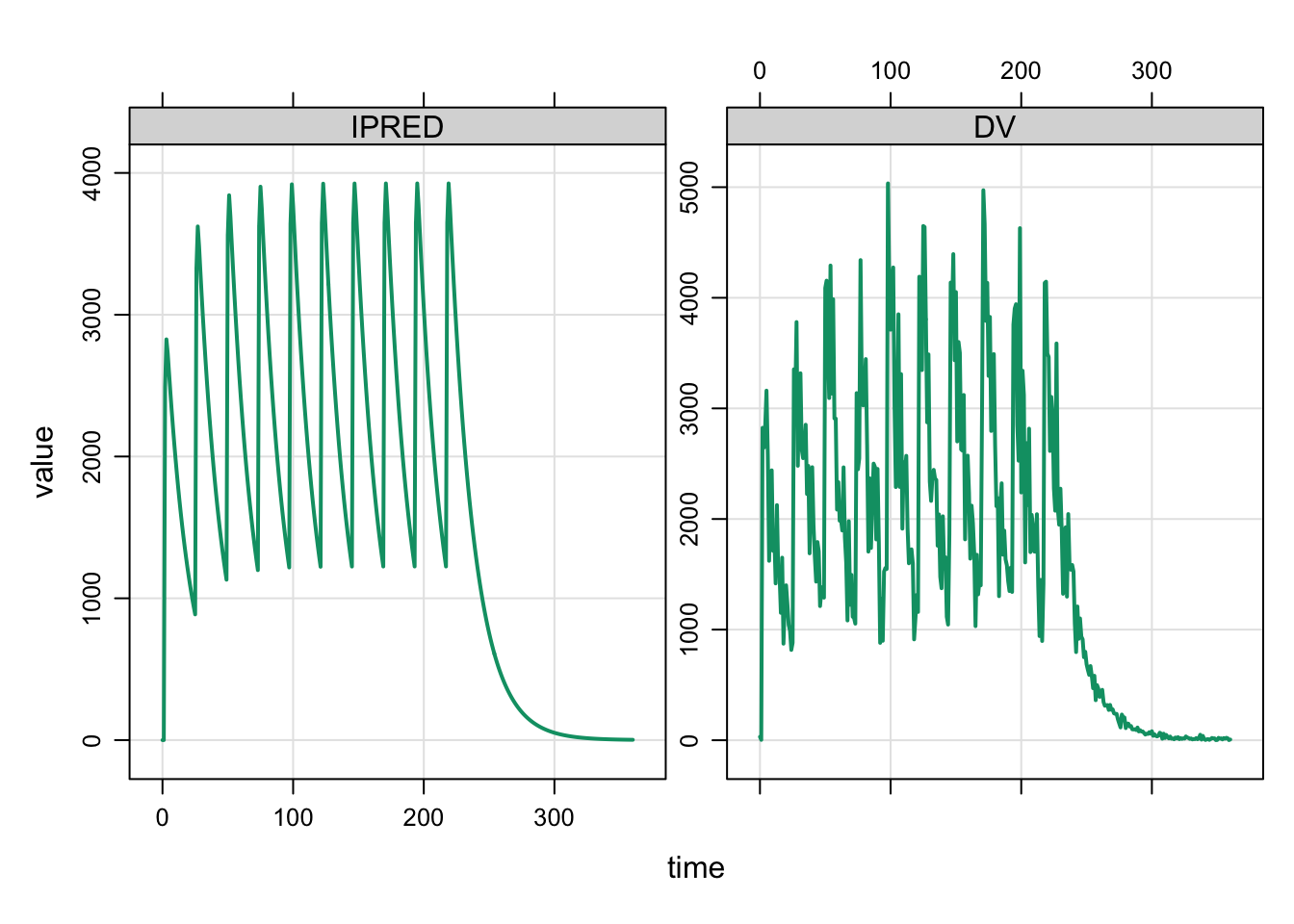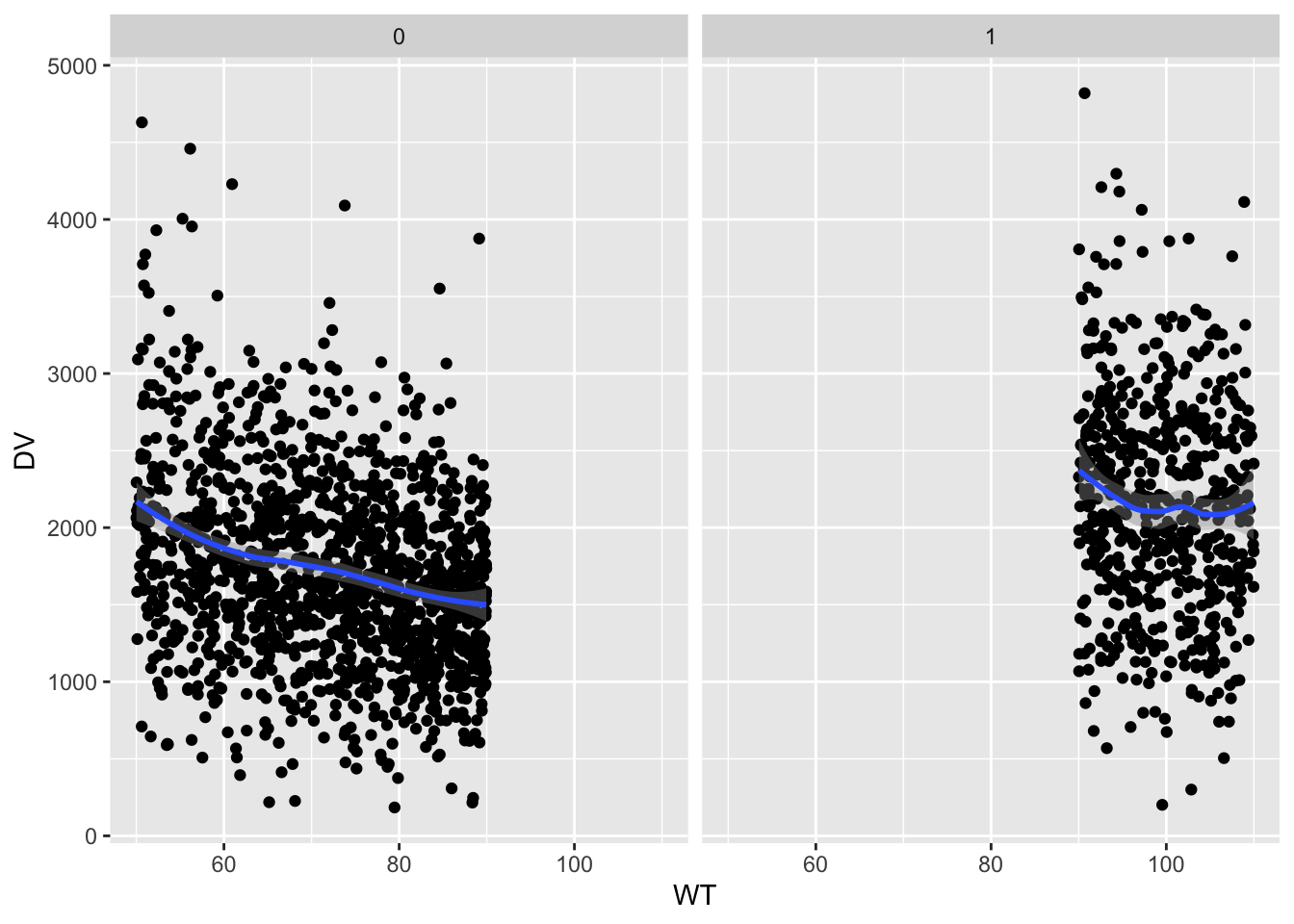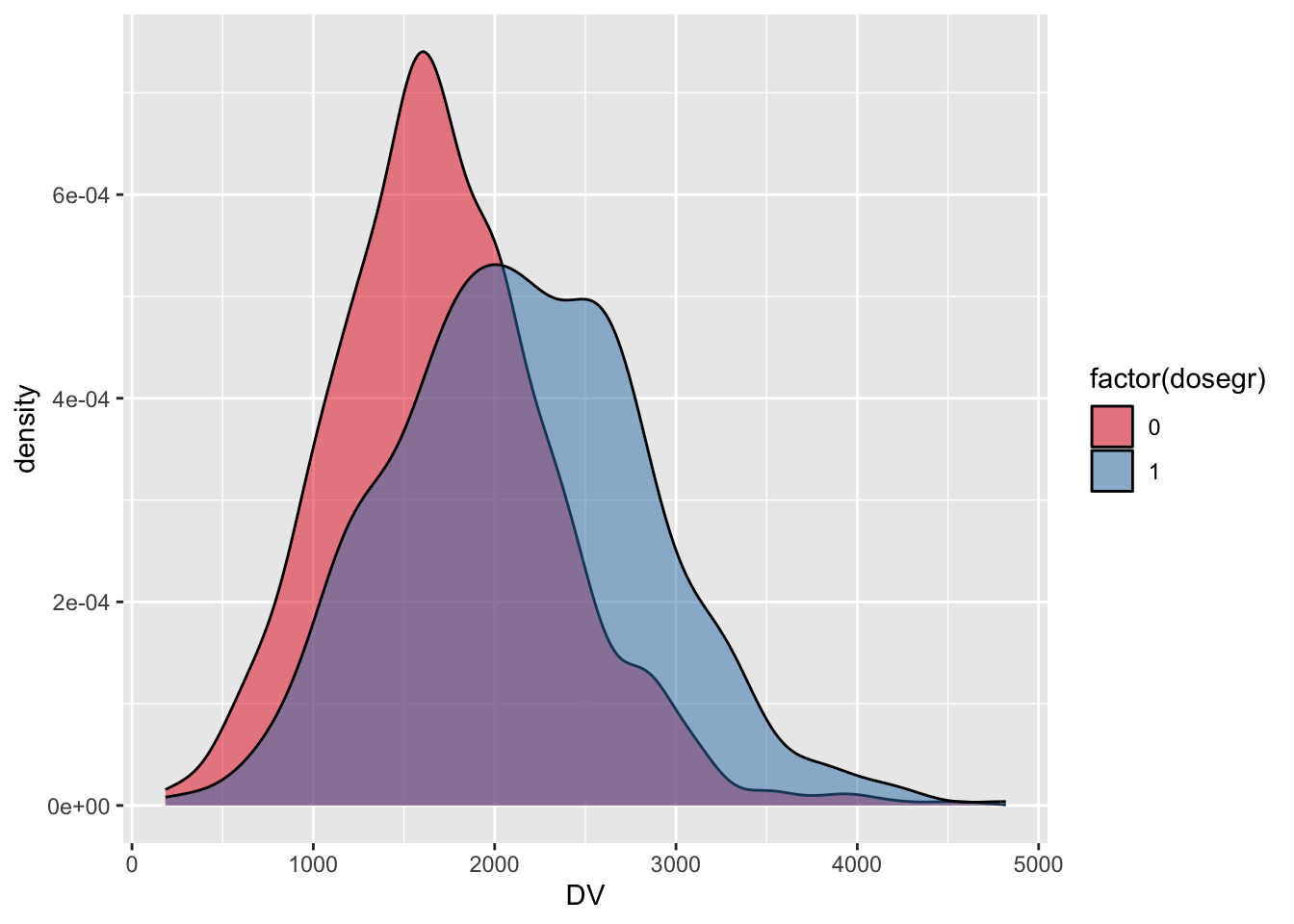# A Complete Example

We had a user who was learning `mrgsolve` ask for a “complete example”. I wasn’t sure what exactly that meant, but I created this example and I’m sharing it today on the blog.

example
Author

Kyle Baron

Published

January 1, 2017

We had a user who was learning `mrgsolve` ask for a “complete example”. I wasn’t sure what exactly that meant, but I created this example and I’m sharing it today on the blog.

This is an invented example to illustrate features and workflow for `mrgsolve`. If you attend one of our training workshops, we work examples using published models to answer real questions you’ll encounter in drug development. So compared to the workshop material, this is a bit contrived. But I wanted to show how you might tackle a problem involving a population model from end to end.

``````library(mrgsolve)
library(dplyr)
library(dmutate)
library(ggplot2)``````

# 1 Population PK model

• One compartment with first order absorption
• There are no ODEs in this model; the system is advanced for each time step through closed-form equations for the amount in each compartment
• Covariates: weight on clearances and volumes, sex on volume
• Log-normally distributed random effects on `CL`, `V`, and `KA`
• Reduced bioavailability fraction for oral doses
• Lag time for oral doses
• Combined additive and proportional error model
• Note: we resimulate residual error variates using `simeps` until the simulated concentration is positive

Here’s the model specification

``````code <- '
\$PARAM TVCL = 1.23, TVV = 35.7, TVKA = 1.3
F1 = 0.82, ALAG = 1.21
WT = 70, SEX = 0

\$MAIN
double CL = TVCL*pow(WT/70,0.75)*exp(ECL);
double V  = TVV*(WT/70)*exp(EV);
double KA = TVKA*exp(EKA);

if(SEX==1) V = V*0.8;

F_GUT = F1;
ALAG_GUT = ALAG;

\$PKMODEL cmt="GUT CENT", depot=TRUE

\$OMEGA @labels ECL EV EKA
0.015 0.2 0.5

0.03 230

\$TABLE
capture IPRED = CENT/(V/1000);

while(DV < 0) {
simeps();
}

\$CAPTURE WT CL
'``````

We use `mcode_cache` here, which caches the model when you compile. If the cache is not invalidated, `mrgsolve` loads from the cache next time rather than re-compiling.

``mod <- mcode_cache("demo", code)``
``Building demo ... done.``

# 2 Input data set

• `N=2000` patients are simulated in this example
• We simulate patient-level weight and sex using the `dmutate` package
• We create a flag in the data set for patients with weight greater than 90 kg
• Patients with weight less than 90 kg get a certain dose while patients with weight greater than 90 kg get a higher dose
• Dosing proceeds Q24H x 10 doses
``````set.seed(33020)
idata <-
data_frame(ID=1:2000) %>%
mutate_random(WT[50,110] ~ rnorm(80,30)) %>%
mutate_random(SEX ~ rbinomial(0.7)) %>%
mutate(dosegr = as.integer(WT > 90))``````
``````Warning: `data_frame()` was deprecated in tibble 1.1.0.
``idata``
``````# A tibble: 2,000 × 4
ID    WT   SEX dosegr
<int> <dbl> <dbl>  <int>
1     1  52.6     0      0
2     2  83.7     1      0
3     3  51.9     1      0
4     4  94.7     0      1
5     5  97.8     1      1
6     6  57.1     1      0
7     7 101.      0      1
8     8  73.0     0      0
9     9  56.3     1      0
10    10  69.7     1      0
# … with 1,990 more rows``````

The dosing elements are implemented through `event` objects.

``````ev1 <- ev(amt=100, ii=24, addl=9)

The `assign_ev` function looks at the `dosegr` column in `idata` and assigns a dosing event sequence (`e1` or `e2`) based on the value of `dosegr`.

``````data <- assign_ev(list(ev1,ev2),idata,"dosegr")

``````  time amt ii addl cmt evid ID
1    0 100 24    9   1    1  1
2    0 100 24    9   1    1  2
3    0 100 24    9   1    1  3
4    0 150 24    9   1    1  4
5    0 150 24    9   1    1  5
6    0 100 24    9   1    1  6``````

NOTE: this is just one way to set up a `data_set` for `mrgsolve`. It might not be the best approach for your problem: maybe it’s too complicated, maybe not complicated enough. See other examples in the blog about creating input data sets or using event objects in your simulations.

# 3 Simulation

• We “carry” (copy) the dose group indicator into the simulated output (`carry_out`)
• Also, we only collect observation records in the output (`obsonly`)
• `mrgsolve` respects the seed you set in `R` using `set.seed` so that results are reproducible
``````set.seed(11009)

out <-
mod %>%
data_set(data) %>%
idata_set(idata) %>%
carry_out(dosegr) %>%
mrgsim(delta=1, end=360, obsonly=TRUE)

out``````
``````Model:  demo
Dim:    722000 x 9
Time:   0 to 360
ID:     2000
ID time dosegr      GUT  CENT    WT     CL IPRED      DV
1:   1    0      0  0.00000  0.00 52.56 0.9589     0   19.92
2:   1    1      0  0.00000  0.00 52.56 0.9589     0   12.37
3:   1    2      0 29.31933 51.23 52.56 0.9589  3236 1954.41
4:   1    3      0  7.97557 68.80 52.56 0.9589  4345 5355.78
5:   1    4      0  2.16955 70.36 52.56 0.9589  4443 3438.75
6:   1    5      0  0.59017 67.74 52.56 0.9589  4278 3689.13
7:   1    6      0  0.16054 64.18 52.56 0.9589  4053 4843.27
8:   1    7      0  0.04367 60.52 52.56 0.9589  3822 5287.74``````

# 4 Output presentation

• For some plots, we use a `plot` method for `mrgsims` objects (the object that is returned from the `mrgsim` function
• For the other plots, it’s really just turning the `mrgsims` object into a `data.frame` and have at it with `ggplot2`
• Other than the quickie `lattice`-based plot method that I only use for quick looks at the output, `mrgsolve` (by design) lets you use packages like `dplyr` or `data.table` or `ggplot` or other great `R` packages that are already out there for summarizing and plotting data
• But notice that `mrgsolve` provides methods for sending the `mrgsims` object directly into a `dplyr` data summary pipeline

This shows the plot method for `mrgsims` objects

``plot(out, IPRED+DV~., subset=ID==10)``The `mrgsims` object can be passed right into `dplyr::filter`

``tr <- filter(out, time==240)``

Simulated day 10 concentration versus patient weight by dose/weight group

``````ggplot(tr, aes(x=WT,y=DV)) +
geom_point() +  facet_wrap(~dosegr) +
geom_smooth(method="loess")``````
```geom_smooth()` using formula = 'y ~ x'``Density plots of day 10 concentrations in the two dose/weight groups

``````ggplot(tr,aes(x=DV,fill=factor(dosegr))) +
geom_density(alpha=0.5) +
scale_fill_brewer(palette="Set1")``````# 5 Summary

This example illustrated how to code a population PK model in `mrgsolve` format, create input data sets with varied dosing and covariate values, simulate, and plot some results. I also hope this example illustrates the design priorities for the `mrgsolve` workflow: we always try to leverage existing functionality available in `R` (such as `dmutate`, `dplyr` and `ggplot`) rather than re-creating our own inside the `mrgsolve` package. This might require you to write some more code, but ultimately it gives greater flexibility to get the simulation that you need for your project.

We regularly do work with models that are more complicated and design simulations that have bigger demands than this example here. We’d be happy to discuss more-complicated applications that you might be needing for your project work. For now we hope this example will give you some ideas how you can add complexity to your simulation project today.